SLPS288E March   2011  – February 2017

PRODUCTION DATA.

1. 1Features
2. 2Applications
3. 3Description
4. 4Revision History
5. 5Specifications
6. 6Application and Implementation
1. 6.1 Application Information
2. 6.2 Typical Application
7. 7Layout
1. 7.1 Layout Guidelines
2. 7.2 Layout Example
8. 8Device and Documentation Support
9. 9Mechanical, Packaging, and Orderable Information

#### Package Options

Refer to the PDF data sheet for device specific package drawings

• DQY|8

## 5 Specifications

### 5.1 Absolute Maximum Ratings

TA = 25°C (unless otherwise noted)(1)
MIN MAX UNIT
Voltage VIN to PGND –0.8 30 V
TG to TGR –8 10 V
BG to PGND –8 10 V
IDM Pulsed current rating(2) 120 A
PD Power dissipation 12 W
EAS Avalanche energy Sync FET, ID = 105 A, L = 0.1 mH 551 mJ
Control FET, ID = 60 A, L = 0.1 mH 180
TJ Operating junction temperature –55 150 °C
Tstg Storage temperature –55 150 °C
Stresses beyond those listed under Absolute Maximum Ratings may cause permanent damage to the device. These are stress ratings only, and functional operation of the device at these or any other conditions beyond those indicated in the Recommended Operating Conditions is not implied. Exposure to absolute-maximum-rated conditions for extended periods may affect device reliability.
Pulse duration ≤ 50 µs. Duty cycle ≤ 0.01%.

### 5.2 Recommended Operating Conditions

TA = 25° (unless otherwise noted)
MIN MAX UNIT
VGS Gate drive voltage 4.5 8 V
VIN Input supply voltage 27 V
ƒSW Switching frequency CBST = 0.1 μF (min) 200 1500 kHz
Operating current 40 A
TJ Operating temperature 125 °C

### 5.3 Thermal Information

TA = 25°C (unless otherwise stated)
THERMAL METRIC MIN TYP MAX UNIT
RθJA Junction-to-ambient thermal resistance (min Cu)(1)(2) 102 °C/W
Junction-to-ambient thermal resistance (max Cu)(1)(2) 50 °C/W
RθJC Junction-to-case thermal resistance (top of package)(2) 20 °C/W
Junction-to-case thermal resistance (PGND pin)(2) 2 °C/W
Device mounted on FR4 material with 1-in2 (6.45-cm2) Cu.
RθJC is determined with the device mounted on a 1-in2 (6.45-cm2), 2-oz (0.071-mm) thick Cu pad on a 1.5-in × 1.5-in
(3.81-cm × 3.81-cm), 0.06-in (1.52-mm) thick FR4 board. RθJC is specified by design while RθJA is determined by the user’s board design.

### 5.4 Power Block Performance

TA = 25° (unless otherwise noted)
PARAMETER TEST CONDITIONS MIN TYP MAX UNIT
PLOSS Power loss(1) VIN = 12 V VGS = 5 V, VOUT = 1.3 V,
IOUT = 25 A, ƒSW = 500 kHz,
LOUT = 0.3 µH, TJ = 25°C
3 W
IQVIN VIN quiescent current TG to TGR = 0 V ,BG to PGND = 0 V 10 µA
Measurement made with six 10-µF (TDK C3216X5R1C106KT or equivalent) ceramic capacitors placed across VIN to PGND pins and using a high-current 5-V driver IC.

### 5.5 Electrical Characteristics

TA = 25°C (unless otherwise stated)
PARAMETER TEST CONDITIONS Q1 CONTROL FET Q2 SYNC FET UNIT
MIN TYP MAX MIN TYP MAX
STATIC CHARACTERISTICS
BVDSS Drain-to-source voltage VGS = 0 V, IDS = 250 μA 30 30 V
IDSS Drain-to-source leakage current VGS = 0 V, VDS = 20 V 1 1 μA
IGSS Gate-to-source leakage current VDS = 0 V, VGS = +10 / –8 100 100 nA
VGS(th) Gate-to-source threshold voltage VDS = VGS, IDS = 250 μA 1 2.1 0.75 1.4 V
ZDS(on)(1) Effective AC on-impedance VIN = 12 V, VGS = 5 V,
VOUT = 1.3 V, IOUT = 20 A,
ƒSW = 500 kHz,
LOUT = 0.3 µH
5 1.2
gƒs Transconductance VDS = 15 V, IDS = 20 A 97 157 S
DYNAMIC CHARACTERISTICS
CISS Input capacitance VGS = 0 V, VDS = 15 V,
ƒ = 1 MHz
1360 1770 2950 3835 pF
COSS Output capacitance 565 735 1300 1690 pF
CRSS Reverse transfer capacitance 19 25 50 65 pF
RG Series gate resistance 1.3 3 0.8 2 Ω
Qg Gate charge total (4.5 V) VDS = 15 V,
IDS = 20 A
8.4 10.9 20 26 nC
Qgd Gate charge gate-to-drain 1.6 3.6 nC
Qgs Gate charge gate-to-source 2.6 4.3 nC
Qg(th) Gate charge at Vth 1.6 2.3 nC
QOSS Output charge VDS = 17 V, VGS = 0 V 9.7 28 nC
td(on) Turnon delay time VDS = 15 V, VGS = 4.5 V,
IDS = 20 A, RG = 2 Ω
7 8 ns
tr Rise time 17 10 ns
td(off) Turnoff delay time 13 33 ns
tƒ Fall time 2.3 4.7 ns
DIODE CHARACTERISTICS
VSD Diode forward voltage IDS = 20 A, VGS = 0 V 0.85 1 0.77 1 V
Qrr Reverse recovery charge Vdd = 17 V, IF = 20 A,
di/dt = 300 A/μs
12.5 32 nC
trr Reverse recovery time 22 28 ns
Equivalent based on application testing. See Equivalent System Performance section for details.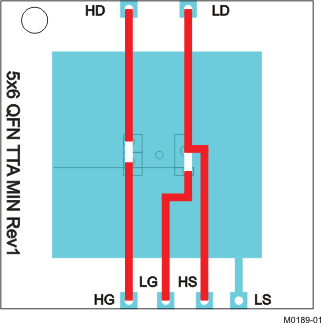Max RθJA = 50°C/W when mounted on 1 in2 (6.45 cm2) of 2-oz (0.071-mm) thick Cu.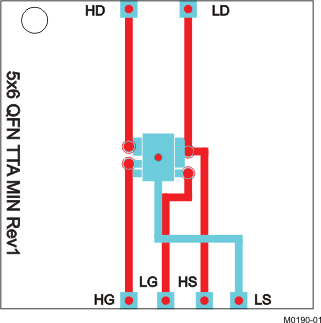Max RθJA = 102°C/W when mounted on minimum pad area of 2-oz (0.071-mm) thick Cu.

### 5.6 Typical Power Block Device Characteristics

TJ = 125°C, unless stated otherwise. The typical power block system characteristic curves Figure 3, Figure 4, and Figure 5 are based on measurements made on a PCB design with dimensions of 4 in (W) × 3.5 in (L) × 0.062 in (H) and 6 copper layers of 1-oz copper thickness. See Application and Implementation for detailed explanation.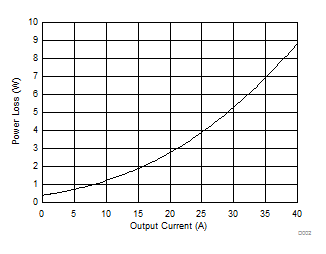VIN = 12 V VGS = 5 V VOUT = 1.3 V ƒSW = 500 kHz LOUT = 0.3 µH
Figure 1. Power Loss vs Output Current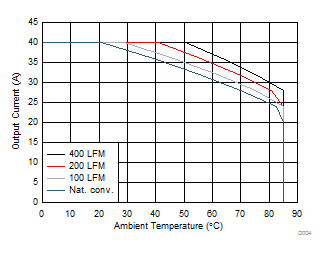VIN = 12 V VGS = 5 V VOUT = 1.3 V ƒSW = 500 kHz LOUT = 0.3 µH
Figure 3. Safe Operating Area (SOA) – PCB Vertical MountVIN = 12 V VGS = 5 V VOUT = 1.3 V ƒSW = 500 kHz LOUT = 0.3 µH
Figure 5. Typical Safe Operating Area (SOA)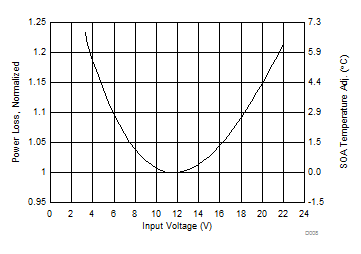VIN = 12 V VOUT = 1.3 V LOUT = 0.3 µH ƒSW = 500 kHz IOUT = 40 A
Figure 7. Normalized Power Loss vs Input Voltage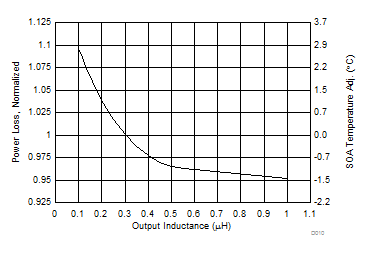VIN = 12 V VGS = 5 V VOUT = 1.3 V ƒSW = 500 kHz IOUT = 40 A
Figure 9. Normalized Power Loss vs Output InductanceVIN = 12 V VGS = 5 V VOUT = 1.3 V ƒSW = 500 kHz LOUT = 0.3 µH
Figure 2. Normalized Power Loss vs Temperature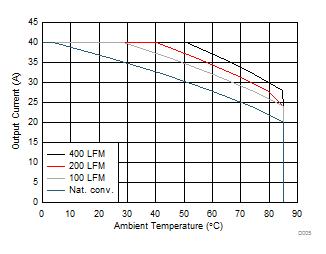VIN = 12 V VGS = 5 V VOUT = 1.3 V ƒSW = 500 kHz LOUT = 0.3 µH
Figure 4. Safe Operating Area (SOA) – PCB Horizontal Mount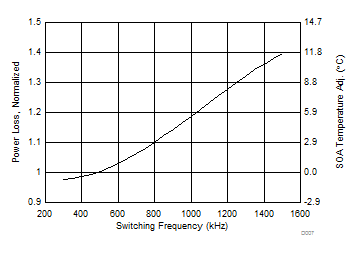VIN = 12 V VGS = 5 V VOUT = 1.3 V LOUT = 0.3 µH IOUT = 40 A
Figure 6. Normalized Power Loss vs Switching Frequency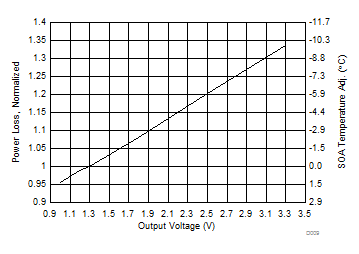VIN = 12 V VGS = 5 V ƒSW = 500 kHz LOUT = 0.3 µH IOUT = 40 A
Figure 8. Normalized Power Loss vs Output Voltage

### 5.7 Typical Power Block MOSFET Characteristics

TA = 25°C, unless stated otherwise.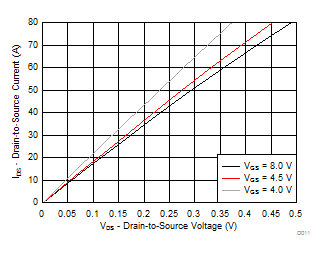Figure 10. Control MOSFET Saturation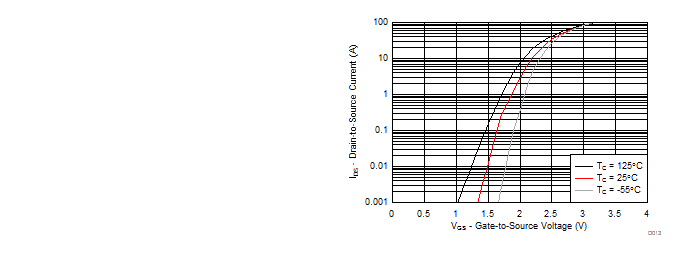VDS = 5 V
Figure 12. Control MOSFET Transfer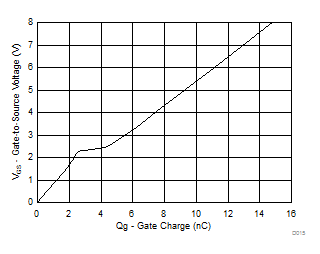ID = 20 A VDD = 15 V
Figure 14. Control MOSFET Gate Charge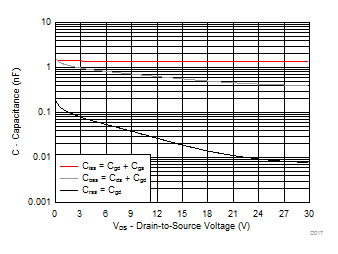ƒ = 1 MHz VGS = 0
Figure 16. Control MOSFET Capacitance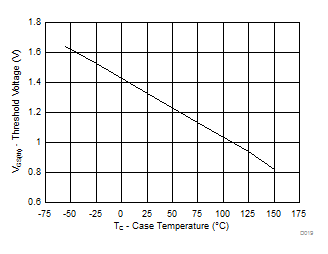ID = 250 µA
Figure 18. Control MOSFET VGS(th)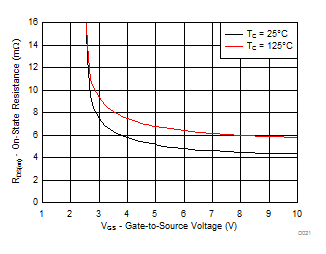Figure 20. Control MOSFET RDS(on) vs VGS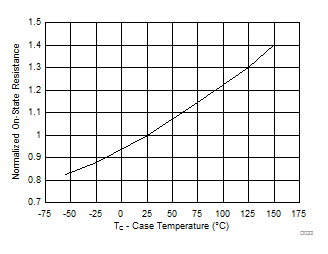ID = 20 A VGS = 8 V
Figure 22. Control MOSFET Normalized RDS(on)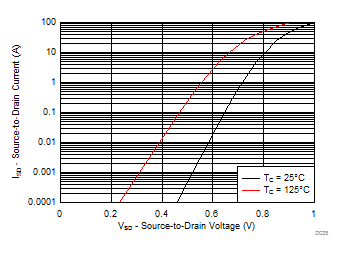Figure 24. Control MOSFET Body Diode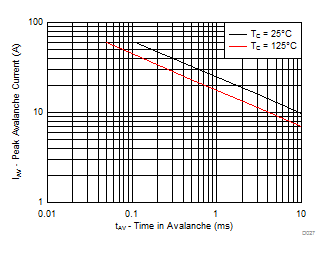Figure 26. Control MOSFET Unclamped Inductive Switching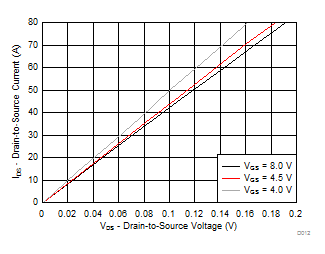Figure 11. Sync MOSFET Saturation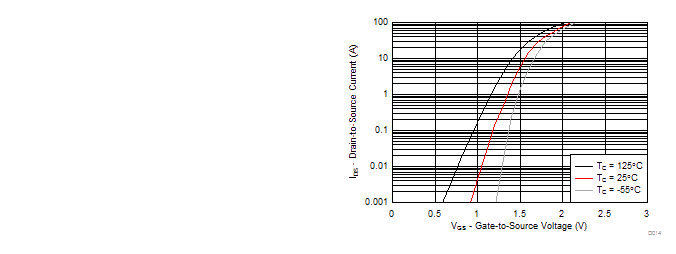VDS = 5 V
Figure 13. Sync MOSFET Transfer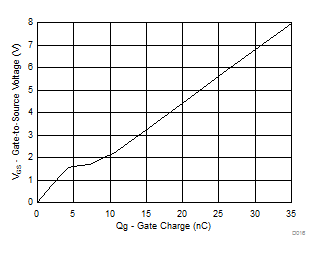ID = 20 A VDD = 15 V
Figure 15. Sync MOSFET Gate Charge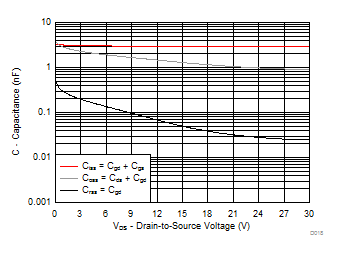ƒ = 1 MHz VGS = 0
Figure 17. Sync MOSFET Capacitance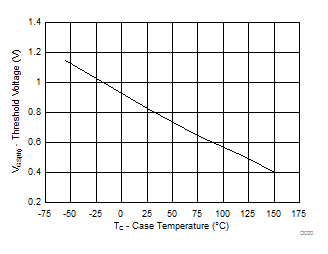ID = 250 µA
Figure 19. Sync MOSFET VGS(th)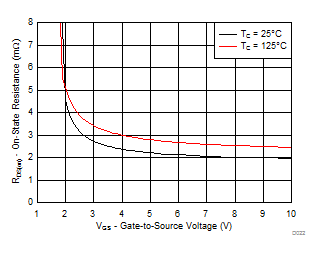Figure 21. Sync MOSFET RDS(on) vs VGS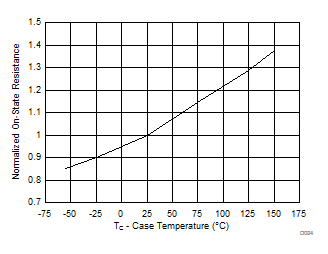ID = 20 A VGS = 8 V
Figure 23. Sync MOSFET Normalized RDS(on)Figure 25. Sync MOSFET Body Diode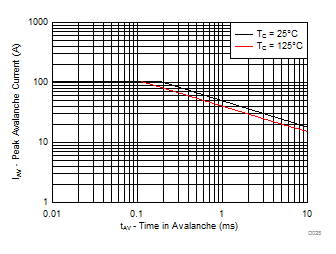Figure 27. Sync MOSFET Unclamped Inductive Switching